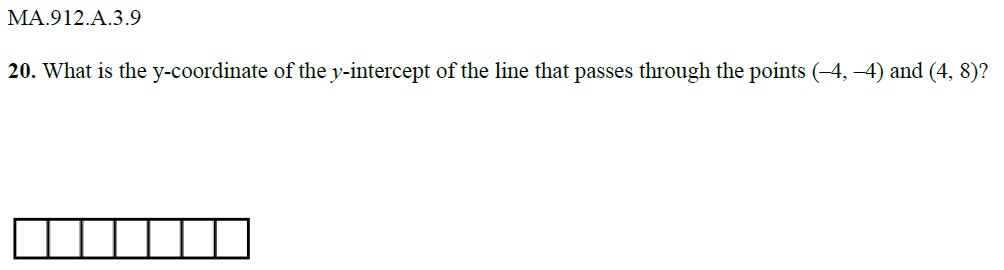Question 20: The Y-Coordinate

Algebra Level 2MA.912.A.3.9 - Determine the slope, x-intercept, and y-intercept of a line given its graph, its equation, or two points on a line.

Algebra 1 EOC Study Guide. How many can you solve correctly?

×